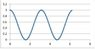# Quantum mechanics relation between p, λ, E, f in a wave

• Samuelriesterer
In summary, the conversation discusses the relationship between momentum and wavelength, and energy and frequency in quantum mechanics. It also covers the calculation of wavelength, wave speed, and mass per unit length of a string, as well as the substitution of momentum and energy in the wave equation using quantum relations. The conversation also touches on the invariance of the Lorentz transformation and the calculation of energy density and power of a wave using the speed of the wave on a string. Finally, it discusses how to calculate the average power during one cycle and the energy density of a wave on a string using the quantum relations. Some additional discussion on the Lorentz transformations for x, t, E, and p is also mentioned.

#### Samuelriesterer

Problem statement, equations, and work done:

In quantum mechanics, there is a relation between momentum and wavelength and between energy and frequency. These are:

##p=\hbar k = \frac{h}{\lambda}##
##E = hf = \hbar \omega##

A wave with an amplitude of 10cm is traveling on a string in the +x direction. The distance between wave crests (tops of oscillations) is 0.5 meters and the string oscillates up and down with a period of 0.10 seconds.

 Calculate the wavelength, write the equation for the wave and calculate the wave speed.

##T=0.1 s##
##f = 10 Hz##
##\lambda = 0.5 m##
##A = 10 cm##
##k = \frac{2\pi}{\lambda} = 12.566##
##v = \frac{\lambda}{T} = 5 m/s##
##\omega = 2\pi f = 62.8 rad/sec##
##y(x,t) = A sin(kx - \omega t) = 10 sin(12.566x – 62.8t)##

 If the tension in the string is 0.01N, determine the mass per unit length of the string.

##F_t = \mu v^2 → \mu = \frac{F_T}{v^2} = \frac{0.01 N}{(5 m/s)^2} = 0.0004 kg/m##

 Use the quantum relations above to substitute momentum and energy for k and ω in the wave equation,; make sure to put the constant in the right places.

##p = \hbar k = \frac{h}{\lambda}##
##E = h f = \hbar \omega##
##k = \frac{p}{\hbar} = \frac{h}{\lambda \hbar}##
##\omega = \frac{E}{\hbar} = \frac{h}{f \hbar}##
##Asin(kx-\omega t) = A sin (\frac{px}{\hbar} - \frac{Et}{\hbar}) = Asin (\frac{hx}{\lambda \hbar} - \frac{ht}{f \hbar})##

 Show that kx-ωt is invariant under the Lorentz transformation. That is, with the E and p substitutions, show that, for an observer moving in the direction of wave travel, transforming x,t,E and p produces the same expression as in the original frame. It is helpful to think of vectors and dot products here.

OK this is where I am stuck.

 Waves carry energy from place to place. To calculate the energy density and power of a wave, start with Power = F•v. Using the note posted to the Canvas Syllabus on the speed of a wave on a string, show that the power is equal to:

##P = F_y \cdot v_y = -F_T tan(\theta) \cdot \frac{\partial y}{\partial t}##

Next, use:
##tan(\theta) = \frac{\partial y}{\partial t}##

to get:
##P = -F_T \frac{\partial y}{\partial x} \frac{\partial y}{\partial t}##

Given y = Asin(kx-ωt), calculate the two partial derivatives and write the full expression for the power. Eliminate FT using v2 = FT/μ and substitute for one of the velocity terms the correct combination of wave parameters ω and k.

Finally for the power, calculate the average power during one cycle by calculating the average of cosine squared over one period.

##f_{average} = <f> = \frac{1}{b-a} \int_a^b f(x) dx##
##<cos^2 (\theta)> = \frac{1}{2\pi} \int 0^{2\pi} cos^2(\theta) d\theta##

The graph attached may be of help in determining that average value.

 Lastly, to get the energy density, use the power as the energy delivered per unit time, so E(Δt) = P Δt and the energy density will be the energy per unit length of string , so Energy density u = E(Δt)/Δx. Carry out these steps to get an expression for the energy density of a wave on a string.

#### Attachments

•Post graph.jpg
13 KB · Views: 431
Samuelriesterer said:
Use the quantum relations above to substitute momentum and energy for k and ω
I think the form wanted is this one:
##A sin (\frac{px}{\hbar} - \frac{Et}{\hbar})##
Samuelriesterer said:
transforming x,t,E and p
What are the Lorentz transformations for those variables?

I think I see something but we haven’t got that far in the Lorentz transformations. So would it be:

##x’ =\gamma (x-vt)##
##t’= \gamma (t - \frac{v}{c^2} x)##
##p=\gamma mv##
##E=\gamma mc^2##

Samuelriesterer said:
I think I see something but we haven’t got that far in the Lorentz transformations. So would it be:

##x’ =\gamma (x-vt)##
##t’= \gamma (t - \frac{v}{c^2} x)##
##p=\gamma mv##
##E=\gamma mc^2##
I guess so. (Never studied either quantum theory or relativity myself.)